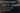# C# 7.2 Stackalloc in safe code## What is the stackalloc keyword in C#?

The keyword 'stackalloc' can be used to allocate a block of memory on the stack. The usage looks like the following:

``int* block = stackalloc int[length];``

The reason you might want to do this is performance related. Allocating on the stack is quite a bit faster and it does not generate any garbage that has to be collected. Additionally it might be better for the cpu cache due to memory locality.

However the above code has a big problem. It uses a pointer and thus needs a unsafe context opening a whole new set of possible problems that could trip you up.

## The safe version

With C# 7.2 and the new 'Span' type that was introduced in the 'System.Memory' nuget package we can now use the 'stackalloc' keyword without having to resort to a unsafe context:

``Span<int> block = stackalloc int[length];``

## The limitations of stackalloc

Now just because you can now use 'stackalloc' in a safe context doesnt mean you should always use it. As 'stackalloc' allocates on the stack the limits of the stack will also be in effect. This means allocating large arrays is probably not the best idea as this can easily lead to a stackoverflow.

Normally the stack size is 1MB but with ASP .NET its only 256/512kb and there might be other flavors around. So even though you could allocate a object that is larger than 85000 bytes and thus normally would need to be allocated on the LOH (Large Object Heap) which is very slow I wouldnt recommend that. Anything larger than a few hundred bytes should be allocated on the heap. At that point the performance difference gets really small (well until you hit the 85000 bytes mark). A simple if else expression could even do this for you at runtime:

``Span<byte> block = length <= 128 ? stackalloc byte[length] : new byte[length];``

However keep in mind that the memory on the stack is freed when the method returns. This means the memory wont be freed at the end of a loop. The result is that the following seemingly fine code will crash with a 'StackOverflowException':

``````var length = 100;
for (int i = 0; i < 100000; i++)
{
Span<byte> block = length <= 128 ? stackalloc byte[length] : new byte[length];
}``````

## The benchmark

As always when talking about performance one should benchmark the results. In the following nchmark I have benchmarked the 3 ways to allocate a array in C#:

``````[Benchmark]
public int HeapAllocatedArray()
{
int[] block = new int[Length];
for (int i = 0; i < block.Length; i++)
{
block[i] = i;
}
var sum = 0;
for (int i = 0; i < block.Length; i++)
{
sum += block[i];
}
return sum;
}

[Benchmark]
public int StackAllocatedArray()
{
Span<int> block = stackalloc int[Length];
for (int i = 0; i < block.Length; i++)
{
block[i] = i;
}
var sum = 0;
for (int i = 0; i < block.Length; i++)
{
sum += block[i];
}
return sum;
}

[Benchmark]
public int UnsafeStackAllocatedArray()
{
unsafe
{
int* block = stackalloc int[Length];

for (int i = 0; i < Length; i++)
{
block[i] = i;
}
var sum = 0;
for (int i = 0; i < Length; i++)
{
sum += block[i];
}
return sum;
}
}``````

And the results of these benchmarks: {{}}

As you can see the difference is getting quite small if you go past a couple of hundred bytes in size. For small array sizes though the difference is quite big.

I have added the unsafe version too but as you can see the performance is pretty much the same as the safe version.